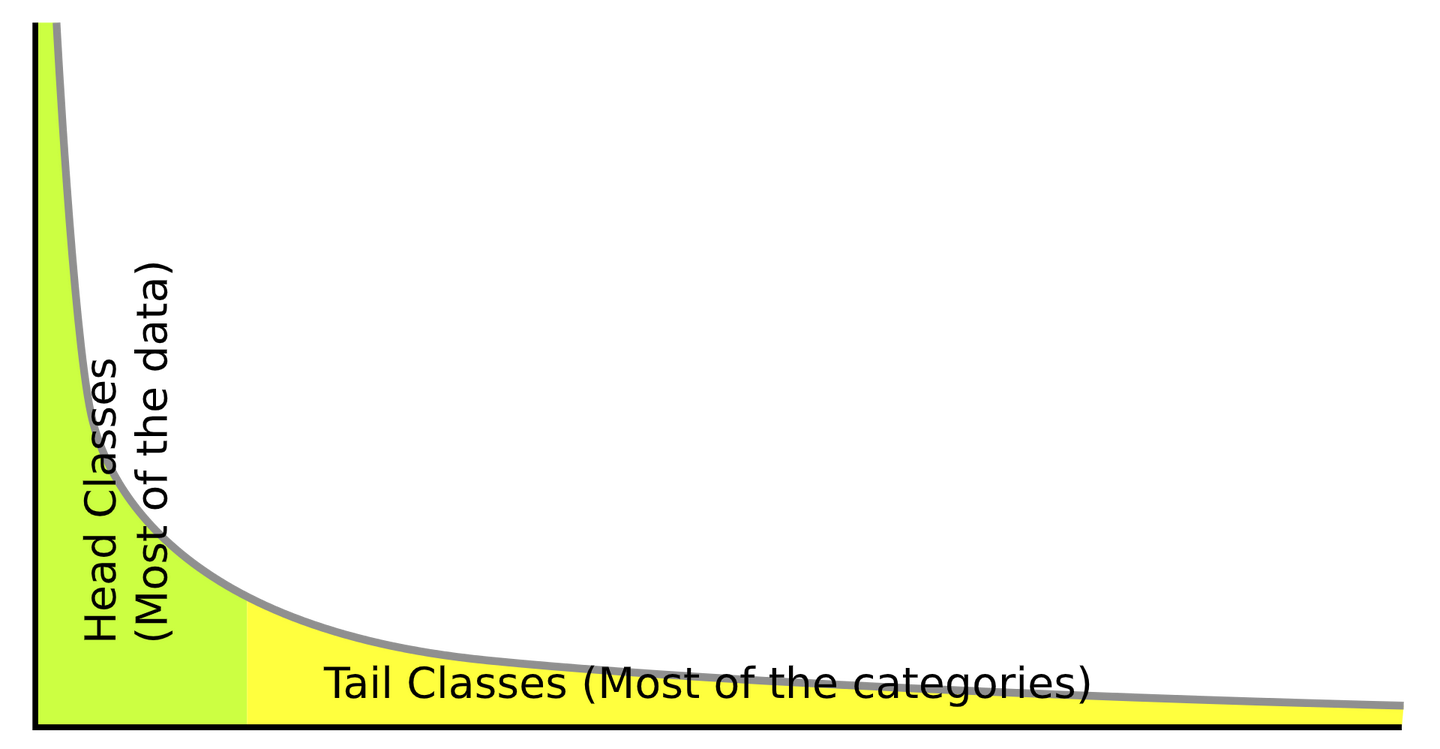# 论文阅读笔记之Long-Tailed Classification by Keeping the Good and Removing the Bad Momentum Causal Effect NIPS，2020

### 背景和动机$$v_{t}=\underbrace{\mu \cdot v_{t-1}}_{\text {momentum }}+g_{t}, \quad \theta_{t}=\theta_{t-1}-l r \cdot v_{t},$$